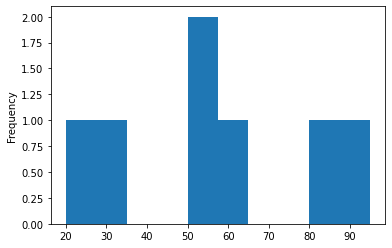In this brief tutorial, we'll see how to map numerical data into categories or bins in Pandas. The process is known also as binning or grouping by data into Categorical.

Typical use case for this operations are:

• financial data
• salaries
• years
• ages
• percentage

We will cover several most interesting examples. To start, create a simple DataFrame with percentage, age, salary columns:

``````data = {'productivity': [80, 20, 60, 30, 50, 55, 95],
'salary': [3500, 1500, 2000, 1000, 2000, 1500, 4000],
'age': [25, 30, 40, 35, 20, 40, 22]}
data_ix = ['Tim', 'Jim', 'Kim', 'Bim', 'Dim', 'Sim', 'Lim']
df_example = pd.DataFrame(data, index=data_ix)
print(df_example.to_markdown())
``````

data:

productivity salary age
Tim 80 3500 25
Jim 20 1500 30
Kim 60 2000 40
Bim 30 1000 35
Dim 50 2000 20
Sim 55 1500 40
Lim 95 4000 22

## Step 1: Map percentage into bins with Pandas cut

Let's start with simple example of mapping numerical data/percentage into categories for each person above. First we need to define the bins or the categories. In this example we will use:

`bins = [0, 20, 50, 75, 100]`

Next we will map the productivity column to each bin by:

``````bins = [0, 20, 50, 75, 100]
df_example['binned'] = pd.cut(df_example['productivity'], bins)
``````

the result is:

binned
Tim (75, 100]
Jim (0, 20]
Kim (50, 75]
Bim (20, 50]
Dim (20, 50]
Sim (50, 75]
Lim (75, 100]

Now we can do value_counts:

binned
(75, 100] 2
(50, 75] 2
(20, 50] 2
(0, 20] 1

## Step 2: Map numeric column into categories with Pandas cut

Now let's group by and map each person into different categories based on number and add new label (their experience/age in the area). Again we need to define the limits of the categories before the mapping. But this we need to have also names for each category:

`bins = [15, 20, 25, 50]`
`category = ['junior', 'mid', 'senior']`

Let see how to map each employee in one of these categories:

``````bins = [15, 20, 25, 50]
category = ['junior', 'mid', 'senior']
df_example['experience'] = pd.cut(df_example['age'], bins, labels=category)
``````

result:

experience
Tim mid
Jim senior
Kim senior
Bim senior
Dim junior
Sim senior
Lim mid

Note 1: that bin labels must be one fewer than the number of bin edges otherwise error is raised:

`ValueError: Bin labels must be one fewer than the number of bin edges`

Note 2: method `cut` will create Categorical column

Note 3: `np.inf` can be used as upper limit

Note 4: `ValueError: bins must increase monotonically` is error shown if the beans are inconsistent

## Step 3: Pandas map numeric column with dictionary

Finally let see how to map existing column to a dictionary and map each value:

``````salary_dict = {1000: 'L1', 1500: 'L2', 2000: 'L3',  2500: 'L4',
2500: 'L5',   3500: 'L6',  4000: 'L7' }
df_example['salary'].map(salary_dict)
``````

The final result is:

salary
Tim L6
Jim L2
Kim L3
Bim L1
Dim L3
Sim L2
Lim L7

Note: Non-Exhaustive Mapping can be handled by:

``````salary_dict = {1000: 'L1', 1500: 'L2', 2000: 'L3',  2500: 'L4'}
df_example['salary'].map(di).fillna(df_example['salary'])
``````

so instead of NaN values the output contains data from the original column(which doesn't have map):

salary
Tim 3500
Jim L2
Kim L3
Bim L1
Dim L3
Sim L2
Lim 4000

## Step 4: Pandas map numeric column with np.select

Finally let's see how to map the values based on selection. The selection is done by: `np.select`. We are going to divide the salaries into two groups:

• low - 0, 2000
• high - 2000, 4000

This is the code:

``````import numpy as np
criteria = [df_example['salary'].between(0, 2000), df_example['salary'].between(2000, 4000)]
values = ['low', 'high']

np.select(criteria, values, 0)
``````

and the output is:

``````['high' 'low' 'low' 'low' 'low' 'low' 'high']
``````

## Step 5: Plotting Categorical data

If you need to plot the data for the productivity then you can do:

`df_example['productivity'].plot(kind='hist')`if you like to plot the mapped bins than you can do:

``````bins = [15, 20, 25, 50]
category = ['junior', 'mid', 'senior']
df_example['experience'] = pd.cut(df_example['age'], bins, labels=category)
df_example['experience'].value_counts().plot(kind='barh')
``````